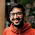Dec 15, 2017

Fraction Brainteaser

Source:
Sent to me by Gaurav Sinha

Problem:
Siddhant writes a Maths test and correctly answers 5 out of 6 Arithmetic questions and 20 out of 28 Geometry questions. In total, Siddhant scores 25 out of 34.

Vaibhav writes another Maths test and correctly answers 20 out of 25 Arithmetic questions and 6 out of 9 Geometry questions. in total, Vaibhav scores 26 out of 34.

Note that
a) Vaibhav scores more than Siddhant
b) Siddhant score better than Vaibhav in both individual topics - 5/6 > 20/25 and 20/28 > 6/9

How is it possible?

1.Score = accuracy * total score
So, for Siddhant higher accuracy (5/6) has lower scoring (6) where as Vaibhav has higher score in more accurate tests(arithmetic)

2.This is Simpson's paradox. Siddhant is better than Vaibhav in each individual subject, but Siddhant's total score is lesser because he attempts more geometry questions, which are harder than arithmetic questions.

See https://en.wikipedia.org/wiki/Simpson%27s_paradox for a more detailed explanation.

3.Score is just the sum of the correct answers. It has nothing to do with fractions.

4.each question of Arithmetic =x, Geometry =y (say)
6x+28y=34, 25x+9y=34
=> x=y=1
Therefore, each has same weightage of 1 mark.
Even though, 5/6 > 20/25 and 20/28 > 6/9 Vaibhav answered more (20+6=26) questions than Siddhant(5+20=25)

5.Let C denote solving problem correctly. Let S denote solving by Siddhant and A denote Arithmetic problem.
Then according to problem P(C | S, A) > P(C | ~S, A) and P(C | S, ~A) > P(C | ~S, ~A) but P(C | S) < P(C | ~S).
This problem is very prominent in statistics. Here the variable A is called the confounding variable, it influences both the independent (S) and dependent variable(C). More can be understood from wiki link on "Confounding".

6.Because a/b+c/d != (a+c)/(b+d)

Fraction Brainteaser

Source: Sent to me by Gaurav Sinha Problem: Siddhant writes a Maths test and correctly answers 5 out of 6 Arithmetic questions and 20...